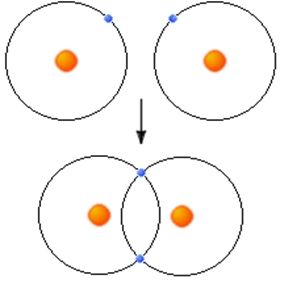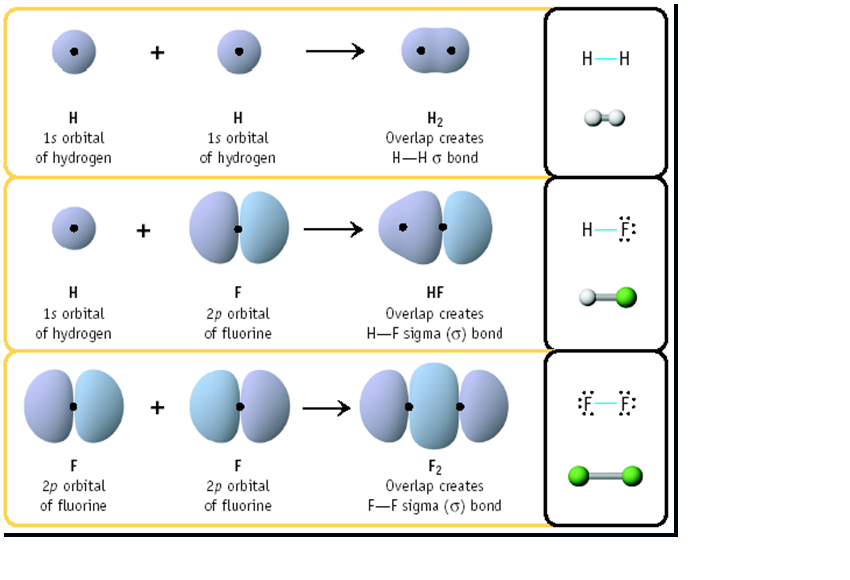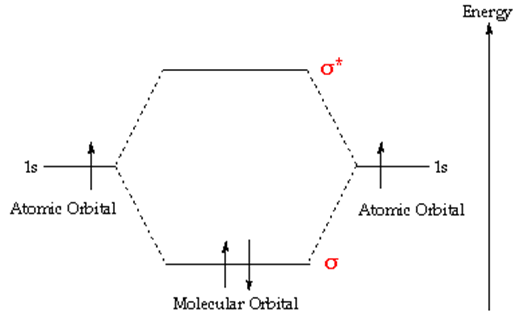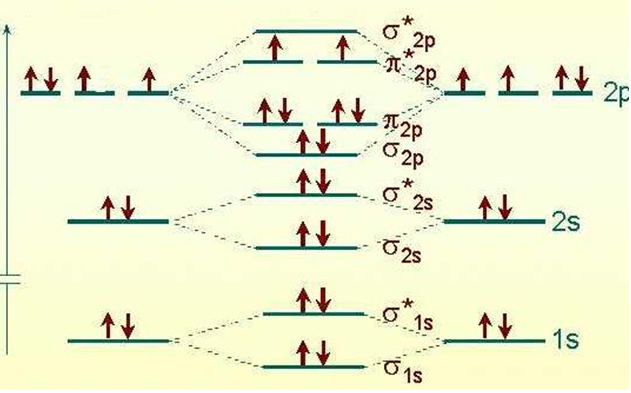# Introduction to Molecular Orbital Theory

##### Introduction

The goal of molecular orbital theory is to describe molecules in a similar way to how we describe atoms, that is, in terms of orbitals, orbital diagrams, and electron configurations.

• Molecules can form bonds by sharing electron
• Two shared electrons form a single bond
• Atoms can share one, two or three pairs of electrons
• forming single, double and triple bonds
• Other types of bonds are formed by charged atoms (ionic) and metal atoms (metallic).
##### Orbital Mixing

When atoms share electrons to form a bond, their atomic orbitals mix to form molecular bonds. In order for these orbitals to mix they must:

• Have similar energy levels.
• Overlap well.
• Be close togetherThis is and example of orbital mixing. The two atoms share one electron each from there outer shell. In this case both 1s orbitals overlap and share their valence electrons.

Examples of Sigma Bond FormationAtomic and Molecular Orbitals :

• In atoms, electrons occupy atomic orbitals, but in molecules they occupy similar molecular orbitals which surround the molecule.
• The two 1s atomic orbitals combine to form two molecular orbitals, one bonding (s) and one anti-bonding (s*).This is an illustration of molecular orbital diagram of H2.
Notice that one electron from each atom is being “shared” to form a covalent bond. This is an example of orbital mixing.MO Diagram for O2

• Each line in the diagram represents an orbital.
• The molecular orbital volume encompasses the whole molecule.
• The electrons fill the molecular orbitals of molecules like electrons fill atomic orbitals in atoms.
• Electrons go into the lowest energy orbital available to form lowest potential energy for the molecule.
• The maximum number of electrons in each molecular orbital is two. (Pauli exclusion principle).
• One electron goes into orbitals of equal energy, with parallel spin, before they begin to pair up. (Hund’s Rule.)

Conclusions

• Bonding electrons are localized between atoms (or are lone pairs).
• Atomic orbitals overlap to form bonds.
• Two electrons of opposite spin can occupy the overlapping orbitals.
• Bonding increases the probability of finding electrons in between atoms.
• It is also possible for atoms to form ionic and metallic bonds.

References

### Feedback is important to us.#### Shiva

I love to write technical articles.

error: Content is protected !!